The angle between two vectors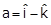and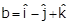is

1.  ${\mathrm{cos}}^{-1}\left(\frac{1}{3}\right)$

2.  ${\mathrm{cos}}^{-1}\left(\frac{-1}{3}\right)$

3.  ${\mathrm{sin}}^{-1}\left(\frac{-1}{3}\right)$

4.  $90°$

Subtopic:  Scalar Product |
Please attempt this question first.
Please attempt this question first.
Launched MCQ Practice Books

Prefer Books for Question Practice? Get NEETprep's Unique MCQ Books with Online Video/Text Solutions via Telegram Bot

The distance x (in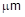) covered by a molecule starting from point A at time t = 0 and stopping at another point B in given by the equation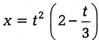The distance between A and B (in) is closed to

1.  10.7

2.  20.7

3.  40.7

4.  50.7

Subtopic:  Differentiation |
Please attempt this question first.
Please attempt this question first.
Launched MCQ Practice Books

Prefer Books for Question Practice? Get NEETprep's Unique MCQ Books with Online Video/Text Solutions via Telegram Bot

A tangential force acting on the top of sphere of mass m kept on a rough horizontal place as shown in figure.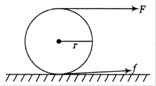If the sphere rolls without slipping, then the acceleration with which the centre of sphere moves, is

1.

2.

3.

4.

Subtopic:  Rolling Motion |
Please attempt this question first.
Please attempt this question first.
Launched MCQ Practice Books

Prefer Books for Question Practice? Get NEETprep's Unique MCQ Books with Online Video/Text Solutions via Telegram Bot

The density of a rod having length l varies as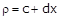, where x is the distance from the left end. The centre of mass is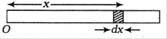1.

2.

3.

4.

Subtopic:  Center of Mass |
Please attempt this question first.
Please attempt this question first.
Launched MCQ Practice Books

Prefer Books for Question Practice? Get NEETprep's Unique MCQ Books with Online Video/Text Solutions via Telegram Bot

One end of a massless spring of constant 100 N/m and natural length 0.5m is fixed and the other end is connected to a particle of mass 0.5 kg lying on a frictionless horizontal table. The spring remains horizontal. If the mass is made to rotate at angular velocity of 2 rad/s, then elongation of spring is

1.  0.1m

2.  10 cm

3.  1 cm

4.  0.01 cm

Subtopic:  Uniform Circular Motion |
Please attempt this question first.
Please attempt this question first.
Launched MCQ Practice Books

Prefer Books for Question Practice? Get NEETprep's Unique MCQ Books with Online Video/Text Solutions via Telegram Bot

A block slides down on an incline of angle 30 ° with an acceleration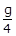. Find the kinetic friction coefficient.

1.  $\frac{1}{2\sqrt{2}}$

2.  $0.6$

3.  $\frac{1}{2\sqrt{3}}$

4.  $\frac{1}{\sqrt{2}}$

Subtopic:  Friction |
Please attempt this question first.
Please attempt this question first.
Launched MCQ Practice Books

Prefer Books for Question Practice? Get NEETprep's Unique MCQ Books with Online Video/Text Solutions via Telegram Bot

Two long straight wires, each carrying an electric current of 5A, are kept parallel to each other at a separation of 2.5cm. Find the magnitude of the magnetic force experiment by 10cm of a wire.

1.  4.0 x 10-4N

2.  3.5 x10-6N

3.  2.0 X 10-5N

4.  2.0 x 10-9N

Subtopic:  Force between Current Carrying Wires |
Please attempt this question first.
Please attempt this question first.
Launched MCQ Practice Books

Prefer Books for Question Practice? Get NEETprep's Unique MCQ Books with Online Video/Text Solutions via Telegram Bot

A wire of resistance 10Q is bent to form a complete circle. Find its resistance between two diametrically opposite point.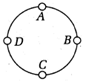1.  $5\mathrm{\Omega }$

2.  $2.5\mathrm{\Omega }$

3.  $1.25\mathrm{\Omega }$

3.  $\frac{10}{3}\mathrm{\Omega }$

Subtopic:  Combination of Resistors |
Please attempt this question first.
Please attempt this question first.
Launched MCQ Practice Books

Prefer Books for Question Practice? Get NEETprep's Unique MCQ Books with Online Video/Text Solutions via Telegram Bot

Find the resistance of a hollow cylindrical Conductor with the inner and outer radii of length 1.0 mm and 2.0 mm respectively. The resistivity of the material is 2.0 x 10-8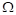m. The length of the conductor is 10 m.

1.  2.1 x 10-32.  1.3 x 10-43.  3.2 x 10-44.  4.6 x 10-2Subtopic:  Derivation of Ohm's Law |
Please attempt this question first.
Please attempt this question first.
Launched MCQ Practice Books

Prefer Books for Question Practice? Get NEETprep's Unique MCQ Books with Online Video/Text Solutions via Telegram Bot

Three equal charges, each having a magnitude of 2.0 x 10-6C, are placed at the three corners of a right angled triangle of sides 3cm, 4cm and 5cm. The force (in magnitude) on the charge at the right angled corner is

1.  50 N

2.  26 N

3.  29 N

4.  45.9 N

Subtopic:  Coulomb's Law |
Please attempt this question first.
Please attempt this question first.
Launched MCQ Practice Books

Prefer Books for Question Practice? Get NEETprep's Unique MCQ Books with Online Video/Text Solutions via Telegram Bot